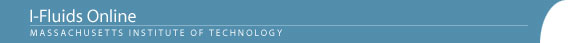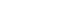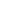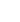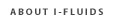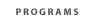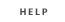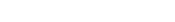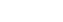2.25: Advanced Fluid Dynamics Section 1: Continuum viewpoint and the equation of motion Section 2: Static Fluids Section 3: Mass Conservation Section 4: Inviscid flow - differential approach Section 5: Control Volume Theorums Section 6: Navier-Stokes equation and viscous flow Section 7: Similarity and dimensional analysis Section 8: Boundary layers, separation and effect on drag/lift Section 9: Vorticity and circulation Section 10: Potential flows; lift, drag and thrust production Section 11: Surface tension and its effect on flows Section 12: Introduction to turbulence Back to 2.25 HomeSection 10: Potential Flow, Lift, and Drag10.1 The occurence of irrotational (potential) flows. The definition of the velocity potential f.
10.2 Incompressible potential flows as solutions of Laplace's equation for the velocity potential with f/∂n specified at the boundaries (the "Neumann problem").
10.3 The equation for the pressure distribution (Bernoulli's integral in terms of f.
10.4 An example: The solution for 2D potential flow over a cylinder. Comparision with experimental data at high Reynolds number, where the flow might be expected to be reasonably "inviscid." Discussion of the pitfalls of potential flow theory.
10.5 [Two-dimensional potential flows. Analytic solutions for simple 2D flows: parallel uniform flow, line source or sink, line vortex. Superposition of simple elemental flows as representations of flows over 2D bodies.]
10.6 Three properties of ideal potential flows around 2D bodies in an infinite stream: (a) The nonexistence of drag (D'Alembert's paradox), (b) the relation between lift and circulation around the body (Kutta-Joukowsky theorem), and (c) the indeterminacy of the circulation in 2D potential flow theory.
10.7 The Kutta condition: an ad hoc criterion, derived from experimental observation, that allows potential flow analysis to be used to establish the circulation (i.e. lift) for a 2D shape with a sharp trailing edge.
10.8 Comments on the fact that viscosity, no matter how "small" it may be in a high Reynolds number flow, is responsible by casing separation for the existence of both lift and drag.
10.9 Qualitative picture of the 3D flow field over a finite lifting surface (wing). Wing-tip vorticies, downwash, etc. Induced drag.
10.10 Overview of lift and drag forces on lifting surfaces.